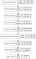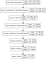# Simple Voice Gender Recognition

When you heard about Gender Recognition you think about Image Processing; however, we can also identify gender using Voice.

# Let’s try!

First, check how we can do Gender Recognition using image.

Then, we can check some audio processing:

After that, we download audio dataset: https://commonvoice.mozilla.org/en/datasets

Dataset contains below labels including the gender (not for all files):

`client_id path sentence up_votes down_votes age gender accent locale segment`

## The ModelUsed model

## The Code

Comment: We need a similar number of female and male audio samples. We split audio files’ spectrogram in blocks of 500ms, these block will be the input of our model. Audio files do not have all the same size, to avoid this problem, we look at the shortest file (for example 2 blocks), and then we randomly took blocks of each audio file in order to use all data we have.

`import csvimport ioimport osimport numpy as npimport randomimport tensorflow as tffrom tensorflow.keras.models import Model, Sequential, load_modelfrom tensorflow.keras.layers import Input, Dense, BatchNormalization, Conv2D, MaxPooling2D, Dropout, Flatten, Reshapefrom tensorflow.keras.layers.experimental import preprocessingimport matplotlib.pyplot as pltmaxData = 5000model_name = "model_gender"frame_length = 1024spect_length = int(frame_length/2+1)step_time = 0.008image_width = 100#100*0.008=800msbatch_size = 64epochs = 15def audioToTensor(filepath):    audio_binary = tf.io.read_file(filepath)    audio, audioSR = tf.audio.decode_wav(audio_binary)    audioSR = tf.get_static_value(audioSR)    audio = tf.squeeze(audio, axis=-1)    frame_step = int(audioSR * step_time)    spectrogram = tf.signal.stft(audio, frame_length=frame_length, frame_step=frame_step)    spect_real = tf.math.real(spectrogram)    spect_real = tf.abs(spect_real)    partsCount = len(spect_real)//image_width    parts = np.zeros((partsCount, image_width, spect_length))    for i, p in enumerate(range(0, len(spectrogram)-image_width, image_width)):        parts[i] = spect_real[p:p+image_width]    return parts, audioSRdef loadDataFromFile(filepath):    dataVoice_male, dataGender_male, dataVoice_female, dataGender_female = [], [], [], []    with open(filepath) as tsvfile:      reader = csv.reader(tsvfile, delimiter='\t')      next(reader)#skip header      for row in reader:        if row == "":            continue        if min(len(dataGender_female), len(dataGender_male))>maxData:            break        filename = row.replace('.mp3', '.wav')        if row == "male":            gender = [1, 0]            dataGender_male.append(gender)            dataVoice_male.append(filename)        else:            gender = [0, 1]            dataGender_female.append(gender)            dataVoice_female.append(filename)    return dataVoice_male, dataGender_male, dataVoice_female, dataGender_femaledataVoice_male, dataGender_male, dataVoice_female, dataGender_female = loadDataFromFile('validated.tsv')print("len(dataGender_male):", len(dataGender_male))print("len(dataGender_female):", len(dataGender_female))dataVoice, dataGender = [], []parts_count = 0min_parts = 100for i in range(0, min(len(dataGender_female), len(dataGender_male))):    dataVoice.append(dataVoice_male[i])    dataVoice.append(dataVoice_female[i])    dataGender.append(dataGender_male[i])    dataGender.append(dataGender_female[i])    parts1 = len(audioToTensor('clips/' + dataVoice_male[i]))    parts2 = len(audioToTensor('clips/' + dataVoice_female[i]))    parts_count += parts1    parts_count += parts2    min_parts = min(min_parts, parts1, parts2)print("parts_count:", parts_count)print("min_parts:", min_parts)class MySequence(tf.keras.utils.Sequence):    def __init__(self, x_voice, y_gender, batch_size, parts_count, min_parts):        self.x_voice, self.y_gender = x_voice, y_gender        self.batch_size = batch_size        self.parts_count = parts_count        self.min_parts = min_parts    def __len__(self):        return (len(self.x_voice)*self.min_parts) // self.batch_size    def __getitem__(self, idx):        batch_x_voice = np.zeros((batch_size, image_width, int(frame_length/2+1)))        batch_y_gender = np.zeros((batch_size, 2))        for i in range(0, batch_size//self.min_parts):            gender = self.y_gender[idx * self.batch_size//self.min_parts + i]            voice, _ = audioToTensor('clips/' + self.x_voice[idx * self.batch_size//self.min_parts + i])            for j in range(0, self.min_parts):                batch_x_voice[i*self.min_parts+j] = random.choice(voice)                batch_y_gender[i*self.min_parts+j] = gender        return batch_x_voice, batch_y_genderif os.path.exists(model_name):    print("Load: " + model_name)    model = load_model(model_name)else:    main_input = Input(shape=(image_width, int(frame_length/2+1)), name='main_input')    x = main_input    x = Reshape((image_width, spect_length, 1))(x)    x = preprocessing.Resizing(image_width//2, spect_length//2)(x)    x = Conv2D(34, 3, activation='relu')(x)    x = Conv2D(64, 3, activation='relu')(x)    x = MaxPooling2D()(x)    x = Dropout(0.1)(x)    x = Flatten()(x)    x = Dense(2, activation='sigmoid')(x)    model = Model(inputs=main_input, outputs=x)    tf.keras.utils.plot_model(model, to_file=model_name+'.png', show_shapes=True)model.compile(optimizer='adam', loss='binary_crossentropy', metrics=['acc'])model.fit(MySequence(dataVoice[0:int(len(dataVoice)*0.8)], dataGender[0:int(len(dataVoice)*0.8)], batch_size, parts_count, min_parts), epochs=epochs, validation_data=MySequence(dataVoice[int(len(dataVoice)*0.8):], dataGender[int(len(dataVoice)*0.8):], batch_size, parts_count, min_parts))model.save(model_name)`

We obtain an accuracy of 0.8785 (on training data) and 0.8750 (on test data).

## Maybe we can improve our model

Since frequencies are one dimension data, we can also try to use Conv1D.Simplified model
`main_input = Input(shape=(image_width, spect_length), name=’main_input’) x = main_input x = BatchNormalization()(x) x = Conv1D(8, 3, activation=’relu’)(x) x = MaxPooling1D()(x) x = AveragePooling1D(pool_size=3, strides=3)(x) x = Dropout(0.1)(x) x = Flatten(name=”flatten”)(x) x = Dense(2, activation=’sigmoid’)(x) model = Model(inputs=main_input, outputs=x)`

We obtain an accuracy of 0.8392 (on training data) and 0.8884 (on test data).

Working in computer science.

## More from Arnaud

Working in computer science.